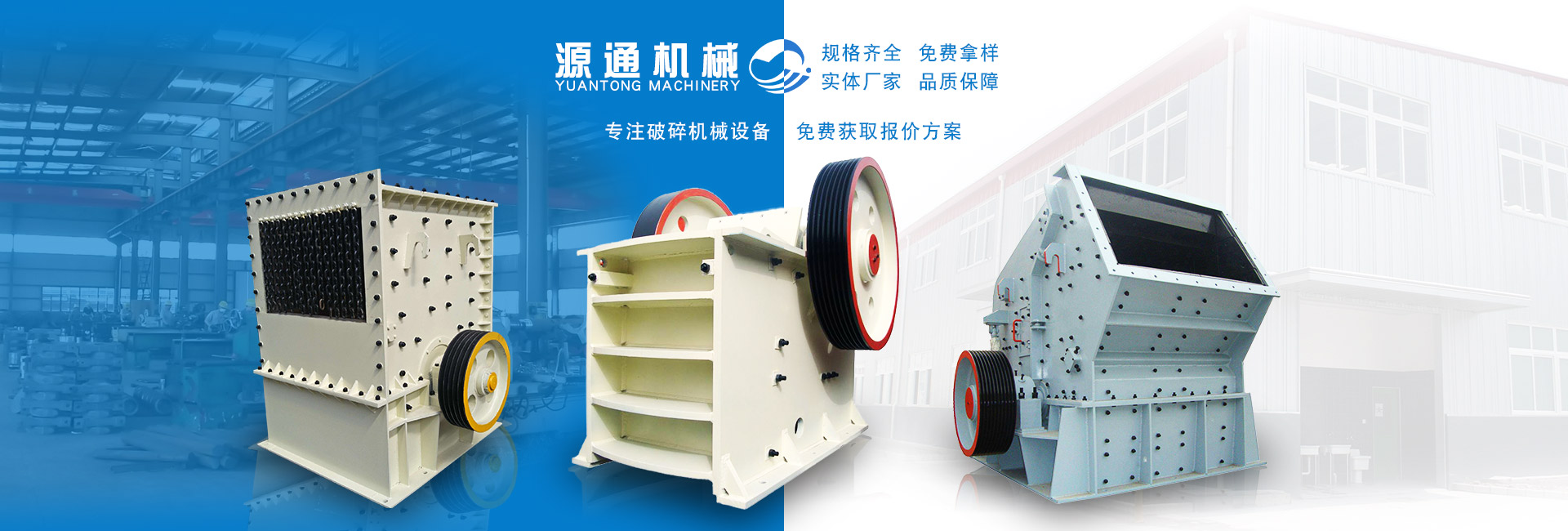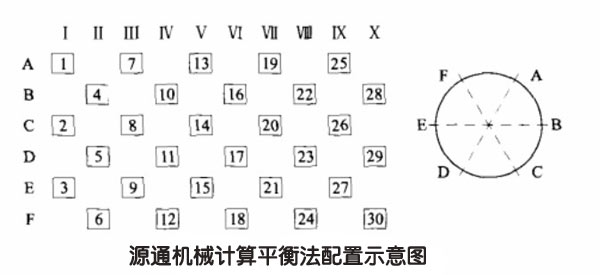•••13838283063

# 锤式破碎机锤头的配重方法

1、计算破碎机锤头平衡法（1）、某一列的5个轴头和其在转子圆周180º方向上对应的5个锤头的总质量分别相等，A5=D5，B5=E5，C5=F5，允许误差正负0.5Kg。

（2）、沿轴线方向上每排3个锤头和其中心对称的3个锤头的总质量分别相等，Ⅰ3=Ⅱ3，Ⅲ3=Ⅳ3，Ⅴ3=Ⅵ3，Ⅶ3=Ⅷ3，Ⅸ3=Ⅹ3，允许误差正负0.5kg。

（3）、每一排上的3个锤头质量也分别相等，1=2=3。4=5=6,7=8=9,10=11=12,13=14=15，16=17=18，19=20=21，22=23=24，25=26=27，28=29=30，允许正负0.25kg。

2、破碎机锤头的现场旋转法

（1）、人工快速转动破碎机皮带轮十几圈，然后让破碎机在惯性状态下停止，找到Ⅰ排较高点，做好标号。重复转动破碎机3-5次，确定Ⅰ排的较佳位置。

（2）、把较重的锤头安装在较高点，较轻的2个锤头分别安装在该排所在圆周上的其他两点。

（3）、然后以同样方法，找出Ⅹ排的较高点，剩余锤头中较重的安装在较高点，较轻的安装在该排所在圆周的其他两点。

（4）、用上述方法依次安装Ⅱ-Ⅸ排、Ⅲ-Ⅷ排、Ⅳ-Ⅶ排和Ⅴ-Ⅵ排，直到安装完毕。

（1）用计算法可在不停机时事先配重好锤头，待更换锤头是，按顺序直接安装，大大节约了维修十几。

（2）现场旋转法必须在停机时进行，维修十几较长，较繁琐。但配重效果比计算法要好。